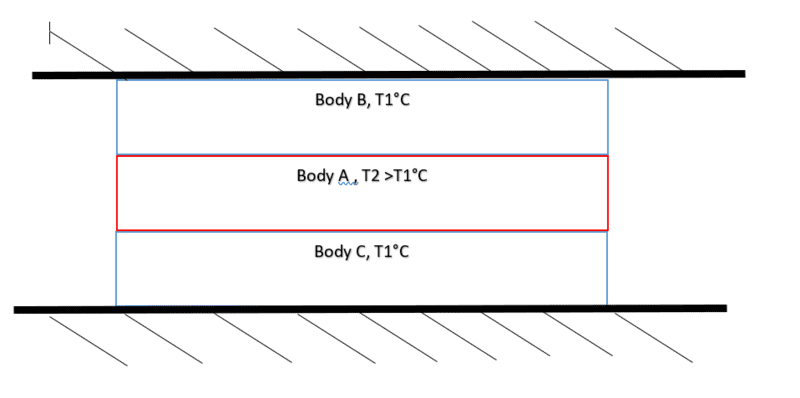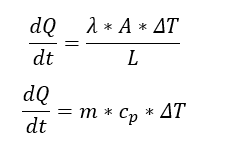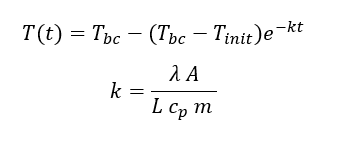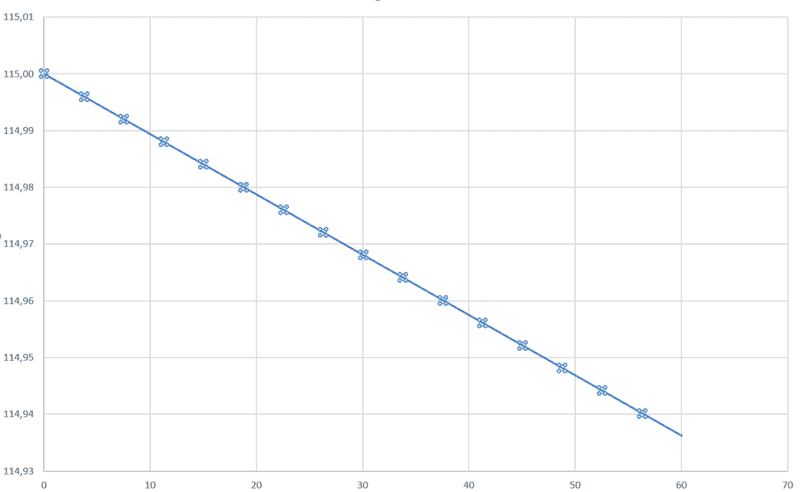# Heat Transfer -- Newton's law of cooling

Hi,

I have been trying to set up a funtcion of the temperature over time and im doubting my results.

In the system i have:Fig 1.

The system is at rest. For simplicty I've considerd the ambient temperature to be same as T1.

By knowing:Fig 2.

yeilds:Fig 3.

In my case B&C is at a constant Temperature of 15°C and Body A 115°C.

The specific heat cond and heat transfer coeff for A is:
0,19 W/m
1700 J/kg

For A:
Length = 2.41m
Width = 2.071m
Depth = 0.01m
Density = 950 kg/m3

For Fig3. i end up with L=0.01m A=0.0207m2 and m = 47.4 kg.

By using the function above and plotting over 60 sec i get.Fig 4

I'm not satisfied with my end result and wondering if anyone can see if there are any obvious errors?

Best Regards

Mikael

#### Attachments

Last edited:

stockzahn
Homework Helper
Gold Member
In my case B&C is at a constant Temperature of 15°C and Body A 115°C.

I'm not quite sure, how your model looks like. Are you trying to find the temperature distribution of your setup or just the transferred heat, i.e. do you assume that all the bodies (A, B, C) have a uniform tempeature at each time? Then what's your length ##L##? Do the bodies B and C are the boundaries (and therefore have the same temperature during your entire calculation)?

Also there are some issues with your units, the conductivity is in ##W/mK## and the heat transfer coefficient in ##W/m^2K##.

One last question: Is this kind of a homework or how did you come up with this scenario?

Yes I assume that the system has a uniform temperature distrubtion.

I want to find the temperature distribution of body A with an initial temp is 115 C. My length is 0.01m. B & C have a constant temp at 15 which can be considered as the boundaries.

Chestermiller
Mentor
Are the temperatures of bodies B and C changing with time? Do you think it is valid to neglect heat conduction within the bodies, and to only use a heat transfer coefficient at their boundaries...or would it be more accurate to assume that heat conduction is occurring and that there is no resistance to heat transfer at the boundaries?

@Chestermiller

There is a heat conduction in the system.

No more heat is generated into body A

Bodies B & C are actively cooling body A with a set point of 15 degrees Celsius. There will be heat conducted to B & C but one can consider that change to negligible.

stockzahn
Homework Helper
Gold Member
@Chestermiller

There is a heat conduction in the system.

No more heat is generated into body A

Bodies B & C are actively cooling body A with a set point of 15 degrees Celsius. There will be heat conducted to B & C but one can consider that change to negligible.

Are the boundaries and the temperature distribution of the Body A you want to assume like in the atttached schematic (for a specific time)?

#### Attachments

@stockzahn

There are no boundaries of body A, yes that is my assumption.

Chestermiller
Mentor
@Chestermiller

There is a heat conduction in the system.

No more heat is generated into body A

Bodies B & C are actively cooling body A with a set point of 15 degrees Celsius. There will be heat conducted to B & C but one can consider that change to negligible.
So, if you are including heat conduction, then are you solving the transient heat conduction equation within body A:
$$\rho C\frac{\partial T}{\partial t}=k\frac{\partial ^2 T}{\partial y^2}$$
where ##\rho## is the density of body A, C is its heat capacity, k is its thermal conductivity, and y is vertical position?

stockzahn
Homework Helper
Gold Member
@stockzahn